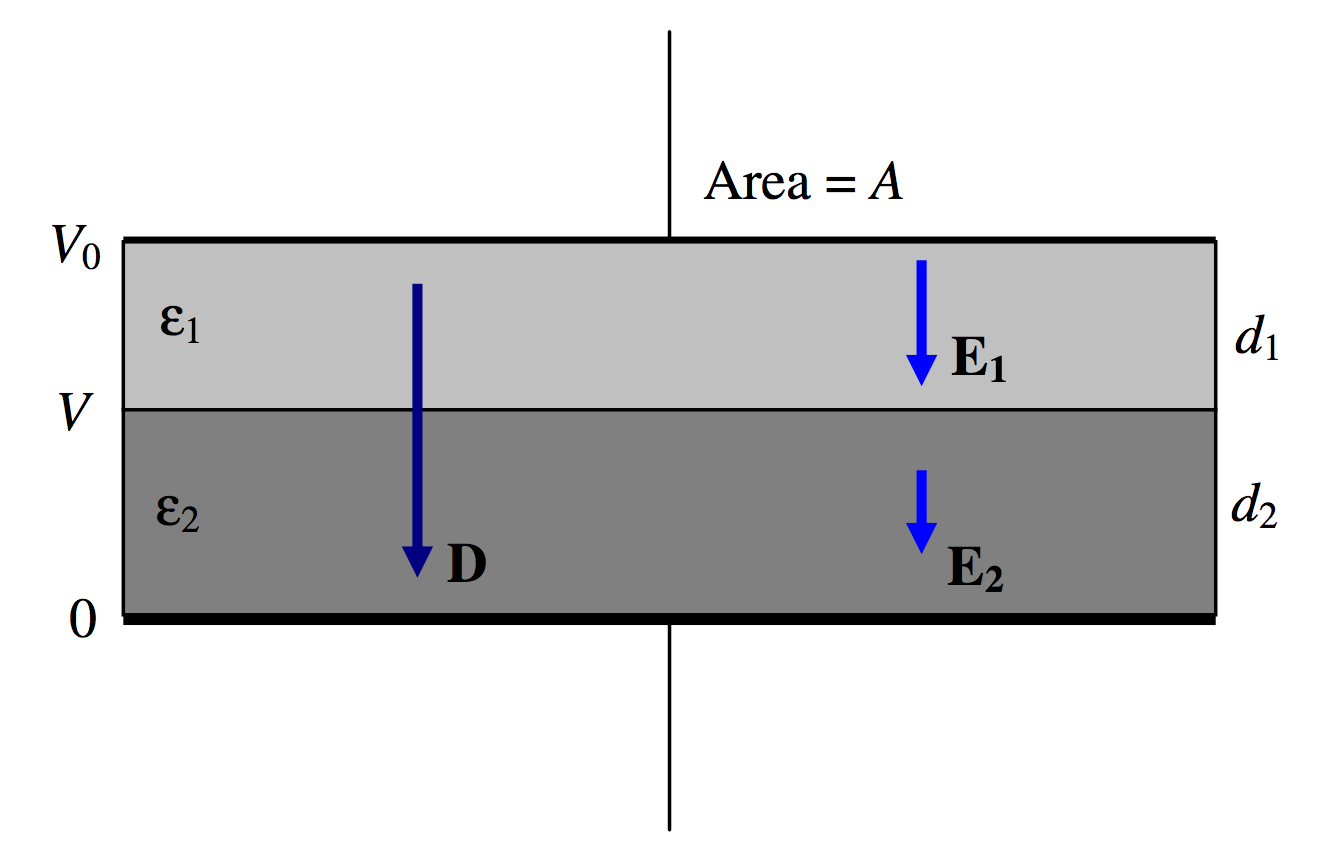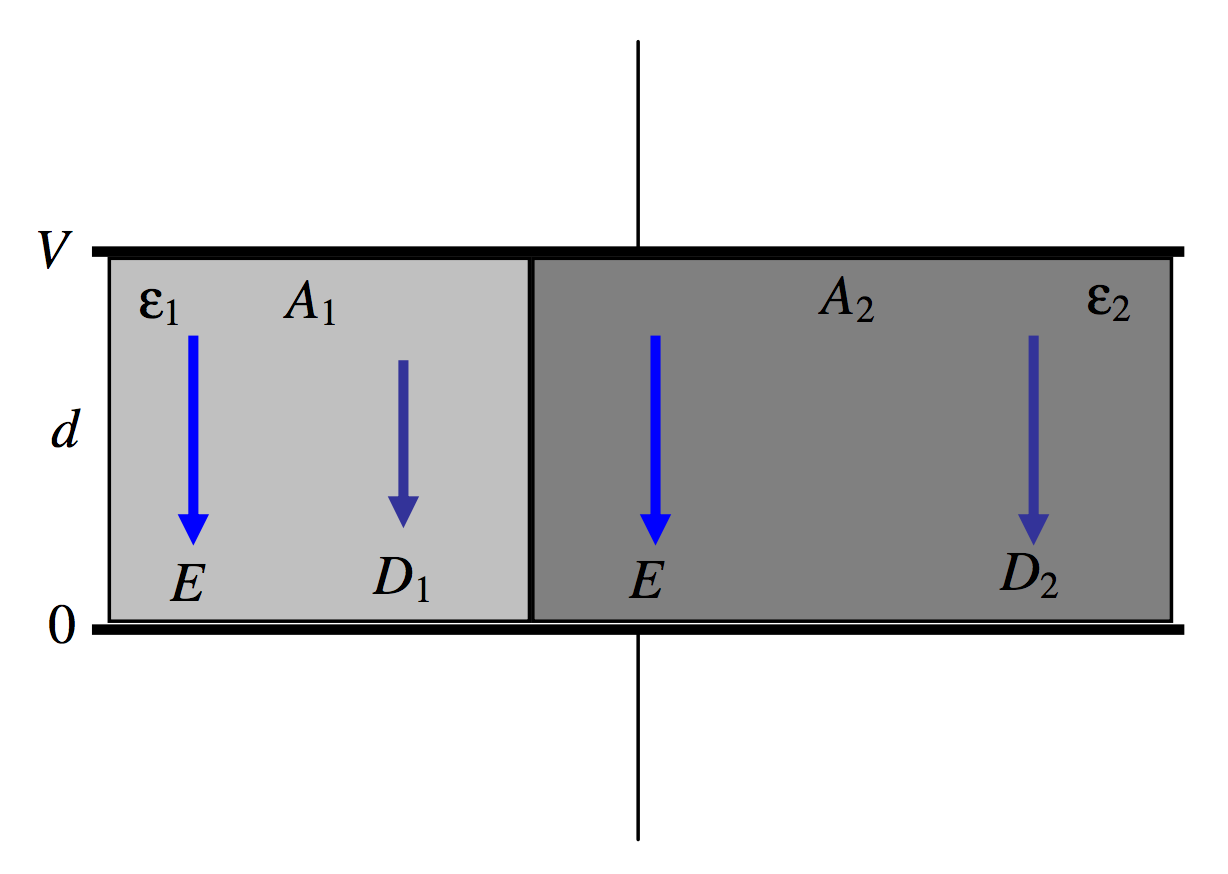$$\require{cancel}$$

5.14: Mixed Dielectrics

•• Jeremy Tatum
• Emeritus Professor (Physics & Astronomy) at University of Victoria

This section addresses the question: If there are two or more dielectric media between the plates of a capacitor, with different permittivities, are the electric fields in the two media different, or are they the same? The answer depends on

1. Whether by “electric field” you mean $$E$$ or $$D$$;
2. The disposition of the media between the plates – i.e. whether the two dielectrics are in series or in parallel.

Let us first suppose that two media are in series (Figure $$V.$$16).$$\text{FIGURE V.16}$$

Our capacitor has two dielectrics in series, the first one of thickness $$d_1$$ and permittivity $$\epsilon_1$$ and the second one of thickness $$d_2$$ and permittivity $$\epsilon_2$$. As always, the thicknesses of the dielectrics are supposed to be small so that the fields within them are uniform. This is effectively two capacitors in series, of capacitances $$\epsilon_1A/d_1 \text{ and }\epsilon_2A/d_2$$. The total capacitance is therefore

$C=\frac{\epsilon_1\epsilon_2A}{\epsilon_2d_1+\epsilon_1d_2}.\label{5.14.1}$

Let us imagine that the potential difference across the plates is $$V_0$$. Specifically, we’ll suppose the potential of the lower plate is zero and the potential of the upper plate is $$V_0$$. The charge $$Q$$ held by the capacitor (positive on one plate, negative on the other) is just given by $$Q = CV_0$$, and hence the surface charge density $$\sigma$$ is $$CV_0/A$$. Gauss’s law is that the total $$D$$-flux arising from a charge is equal to the charge, so that in this geometry $$D = \sigma$$, and this is not altered by the nature of the dielectric materials between the plates. Thus, in this capacitor, $$D = CV_0/A = Q/A$$ in both media. Thus $$D$$ is continuous across the boundary.

Then by application of $$D = \epsilon E$$ to each of the media, we find that the $$E$$-fields in the two media are $$E_1$$=$$Q$$/$$(\epsilon_1A$$) and $$E_2$$=$$Q$$/$$(\epsilon_2A$$), the $$E$$-field (and hence the potential gradient) being larger in the medium with the smaller permittivity.

The potential V at the media boundary is given by $$V/d_2=E_2$$. Combining this with our expression for $$E_2$$, and $$Q = CV$$and Equation \ref{5.14.1}, we find for the boundary potential:

$V=\frac{\epsilon_1d_2}{\epsilon_2d_1+\epsilon_1d_2}V_0.\label{5.14.2}$

Let us now suppose that two media are in parallel (Figure $$V.$$17).$$\text{FIGURE V.17}$$

This time, we have two dielectrics, each of thickness $$d$$, but one has area $$A_1$$ and permittivity $$\epsilon_1$$ while the other has area $$A_2$$ and permittivity $$\epsilon_2$$. This is just two capacitors in parallel, and the total capacitance is

$C=\frac{\epsilon_1A_1}{d}+\frac{\epsilon_2A_2}{d}\label{5.14.3}$

The $$E$$-field is just the potential gradient, and this is independent of any medium between the plates, so that $$E = V/d$$. in each of the two dielectrics. After that, we have simply that $$D_1=\epsilon_1E \text{ and }D_2=\epsilon_2E$$. The charge density on the plates is given by Gauss’s law as $$\sigma = D$$, so that, if $$\epsilon_1 < \epsilon_2$$, the charge density on the left hand portion of each plate is less than on the right hand portion – although the potential is the same throughout each plate. (The surface of a metal is always an equipotential surface.) The two different charge densities on each plate is a result of the different polarizations of the two dielectrics – something that will be more readily understood a little later in this chapter when we deal with media polarization.

We have established that:

1. The component of $$\textbf{D}$$ perpendicular to a boundary is continuous;
2. The component of $$\textbf{E}$$ parallel to a boundary is continuous.

In Figure $$V.$$18 we are looking at the $$D$$-field and at the $$E$$-field as it crosses a boundary in which $$\epsilon_1 < \epsilon_2$$. Note that $$D_y$$ and $$E_x$$are the same on either side of the boundary. This results in:

$\frac{\tan \theta_1}{\tan \theta_2}=\frac{\epsilon_1}{\epsilon_2}.\label{5.14.4}$$$\text{FIGURE V.18}$$